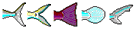Values shown below are defaults; and K is an estimate from the Family where the species belongs. Please double-check, replace with better values as appropriate, and 'Recalculate'.
 About this page...Life History Data on Nematistius pectoralis
Roosterfish
Family: Nematistiidae                           Roosterfish
Max. length (Lmax): = cm
L infinity (Linf): =    s.e. - cm
L maturity (Lm):     s.e. - cm
Estimated from Linf.
L max. yield (Lopt):    s.e. - cm
Estimated from Linf.
K: /year
 Estimate K from Linf, Lm and tm: Lm =    cm tm = years Estimate K from Linf and tmax: tmax = years Enter Linf and K: Linf =    cm SL FL TL WD NG OT K = /year
to: years       Estimated from Linf and K.
Natural mortality (M):   s.e. - /year
°C
Life span (approx.):     s.e. -    years      Estimated from Linf., K and to.
Generation time:     s.e. -    years       Estimated from Lopt, Linf., K and to.
Age at first maturity (tm):     s.e. -    years       Estimated from Lm, Linf., K and to.
Length-weight: cm => (wet weight)
W = * L ^

Length-weight data
Nitrogen & protein:
 Weight (g) => whole-body nitrogen (N) (g) => whole-body crude protein (g)
Relative Yield per Recruit (Y'/R): Estimate Y'/R from M/K, Lc/Linf and E.
Lc = cm    E =   /year
Emsy /year    Eopt /year
Fmsy /year    Fopt /year
Exploitation: Z=
F=
E=
Estimate Z, F, E from Lc, Lmean, Linf, K, M
Lc = cm
Lmean = cm
Resilience / productivity:
Vulnerable to extinction if decline in biomass or numbers exceeds threshold over the longer of 10 years or 3 generations.
Intrinsic rate of increase (rm): /year Lr = cm
Estimated from Fmsy at Lc = length of recruitment (Lr).
Main food: mainly animals (troph. 2.8 and up)
Trophic level: 4.5     +/- s.e. 0.80          Estimated from food data.           Food
Food consumption (Q/B): times the body weight per year Enter Winf, temperature, aspect ratio (A), and food type to estimate Q/B
Winf = g      Temp. = °C
A =Detrivore Herbivore Omnivore Carnivore
Note: The estimates are derived from default values taken from FishBase and will thus not be appropriate for every population. You can change these values and recalculate the life history parameters. Back to Search
Species summary
Life History Data created by: Eli, 01.06.99, php script by: kbanasihan, 11.02.10, last modified by kbanasihan, 11.11.10